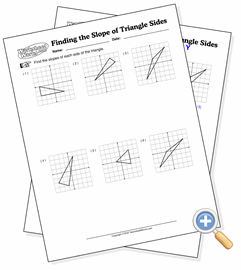# Finding the Slope of Triangle SidesFind the slopes of the sides of triangles on graphs.

In this worksheet, each grid has a triangle. The student is asked to find the slopes of each side of the triangle. The vertices of the triangle have integer coordinates, so the computed slopes come out clean.